# Both17 and 34 are Factors of 1734 and Help It Make Sum-Difference

### Today’s Puzzle:

1734 has only 6 factor pairs, but two of those pairs will solve a Sum-Difference puzzle.

Let’s start with the first puzzle below. Can you find a factor pair of 6 that adds up to 5 and another one that subtracts to 5? If you can, then you have solved the first puzzle. The second puzzle is really just a multiple of the first puzzle. 5 × what = 85? Multiply the numbers you write in the first puzzle by that same number, and you will have the numbers needed to solve the second puzzle. You can also look at the factor pairs in the next section to help you find the needed factors that sum or subtract to 85.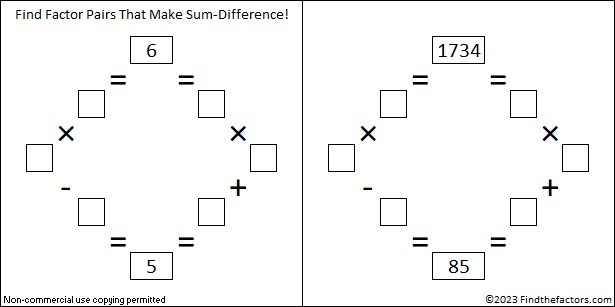### Factors of 1734:

Because 17×2=34, their concatenation, 1734, is divisible by three. All of the following concatenated numbers are also divisible by three: 12, 24, 36, 48, 510, 612, 714, 816, 918, 1020, 1122, 1224, 1326, 1428, 1530, 1632, 1734, 1836, 1938, and so forth.

• 1734 is a composite number.
• Prime factorization: 1734 = 2 × 3 × 17 × 17, which can be written 1734 = 2 × 3 × 17².
• 1734 has at least one exponent greater than 1 in its prime factorization so √1734 can be simplified. Taking the factor pair from the factor pair table below with the largest square number factor, we get √1734 = (√289)(√6) = 17√6.
• The exponents in the prime factorization are 1, 1, and 2. Adding one to each exponent and multiplying we get (1 + 1)(1 + 1)(2 + 1) = 2 × 2 × 3 = 12. Therefore 1734 has exactly 12 factors.
• The factors of 1734 are outlined with their factor pair partners in the graphic below.### More About the Number 1734:

Did you notice that both 17 and 34 are factors of 1734?

From OEIS.org we learn that 1734 has three factors that have 8 as one of their digits, 867, 578, and 289. What do you get when you add those factors together?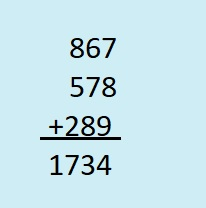1734 is the hypotenuse of two Pythagorean triples:
816-1530-1734, which is (8-15-17) times 102. and
966-1440-1734, which is 6 times (161-240-289).

1734 looks interesting in some other bases:
It’s 600 in base 17 because 6(17²) = 1734, and
it’s 369 in base 23 because 3(23²) + 6(23) + 9(1) = 1734.

What is the greatest common factor of 1734 and 2023? What is the least common multiple? The easiest way to know is to look at their prime factorizations.
1734 = 2·3·17²,
2023 = 7·17².
The greatest common factor is what they have in common: 17² = 289.
The least common multiple is 2·3·7·17² = 12138.

# 1733 and Its Prime Number Substrings

### Today’s Puzzle:

Use logic to write each number from 1 to 12 in both the first column and the top row so that those numbers are the factors of the given clues.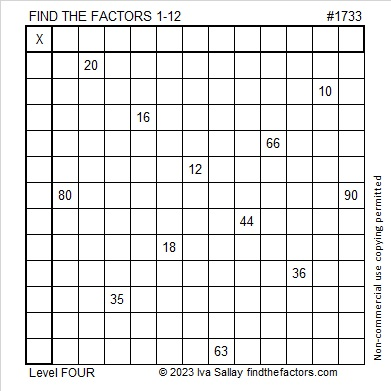### Factors of 1733:

• 1733 is a prime number.
• Prime factorization: 1733 is prime.
• 1733 has no exponents greater than 1 in its prime factorization, so √1733 cannot be simplified.
• The exponent in the prime factorization is 1. Adding one to that exponent we get (1 + 1) = 2. Therefore 1733 has exactly 2 factors.
• The factors of 1733 are outlined with their factor pair partners in the graphic below.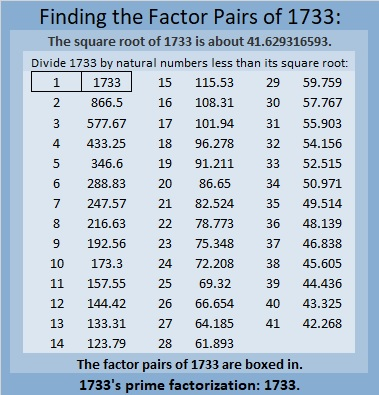How do we know that 1733 is a prime number? If 1733 were not a prime number, then it would be divisible by at least one prime number less than or equal to √1733. Since 1733 cannot be divided evenly by 2, 3, 5, 7, 11, 13, 17, 19, 23, 29, 31, 37, or 41, we know that 1733 is a prime number.

### More About the Number 1733:

1733 is the sum of two squares:
38² + 17² = 1733.

1733 is the hypotenuse of a Pythagorean triple:
1155-1292-1733 calculated from 38² – 17², 2(38)(17), 38² + 17².

Here’s another way we know that 1733 is a prime number: Since its last two digits divided by 4 leave a remainder of 1, and 38² + 17² = 1733 with 38 and 17 having no common prime factors, 1733 will be prime unless it is divisible by a prime number Pythagorean triple hypotenuse less than or equal to √1733. Since 1733 is not divisible by 5, 13, 17, 29, 37, or 41, we know that 1733 is a prime number.

1733 is also the difference of two squares:
867² – 866² = 1733.
That means 1733 is also the short leg of the Pythagorean triple calculated from
867² – 866², 2(867)(866), 867² + 866².

1733 is a palindrome in three bases:
It’s 2101012 in base 3 because
2(3⁶) +1(3⁵) + 0(3⁴) + 1(3³) + 0(3²) +1(3¹) + 2(3⁰) = 1733,
It’s 565 in base 18 because 5(18²) + 6(18) + 5(1) = 1733, and
it’s 4F4 in base 19 because 4(19²) + 15(19) + 4(1) = 1733.

The next fact I learned from Twitter:

What prime numbers can be made with the digits of 1733?
3, 7, 13, 17, 31, 37, 71, 73, 137, 173, 313, 317, 331, 337, 373, 733, 1733.

The numbers in red are the 6 prime numbers that are substrings of 1733. I made a gif showing those 6 primes:make science GIFs like this at MakeaGif
1733 is quite a fascinating number!

# 1732 Is a Thousand Times More Than…

### Today’s Puzzle:

If you know the common factor of 50 and 35 that will put only numbers from 1 to 12 in the first column and the top row of this multiplication table puzzle, then you will have completed the first step in solving the puzzle. Afterward, just work from the top of the puzzle to the bottom filling in factors as you go. That’s how you solve these level-three puzzles.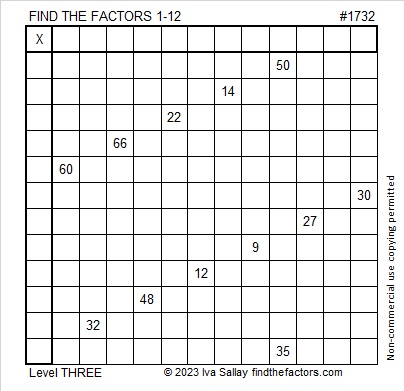### Factors of 1732:

• 1732 is a composite number.
• Prime factorization: 1732 = 2 × 2 × 433, which can be written 1732 = 2² × 433.
• 1732 has at least one exponent greater than 1 in its prime factorization so √1732 can be simplified. Taking the factor pair from the factor pair table below with the largest square number factor, we get √1732 = (√4)(√433) = 2√433.
• The exponents in the prime factorization are 2 and 1. Adding one to each exponent and multiplying we get (2 + 1)(1 + 1) = 3 × 2 = 6. Therefore 1732 has exactly 6 factors.
• The factors of 1732 are outlined with their factor pair partners in the graphic below.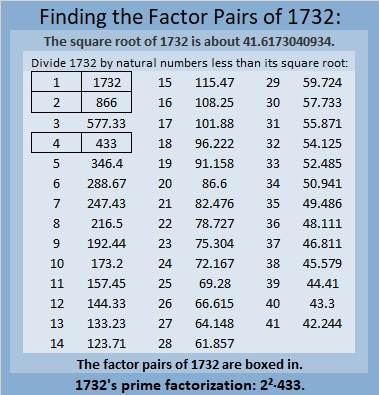### More About the Number 1732:

1732 is the sum of two squares:
34² + 24² = 1732.

1732 is the hypotenuse of a Pythagorean triple:
580-1632-1732, calculated from 34² – 24², 2(34)(24), 34² + 24².
It is also 4 times (145-408-433).

1732 is a palindrome in base 15 because
7(15²) + 10(15) + 7(1) = 1732.

I remember one of my college professors telling his class that
√2 is about 1.4, and Valentine’s day is February 14,
√3 is about 1.7, and Saint Patrick’s day is March 17.

That’s how I remember those values, but this tweet reminded me that 1732 is a thousand times more than the square root of three rounded to three decimal places. It also makes a reference to the square root of two.

# 1731: The Sum of the Squares of Three Consecutive Prime Numbers

### Today’s Puzzle:

Write the numbers from 1 to 12 in both the first column and the top row so that the given clues are the products of the numbers you write.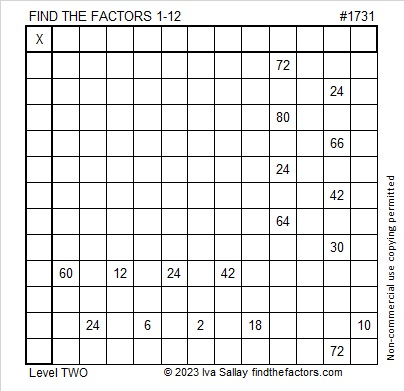### Factors of 1731:

• 1731 is a composite number.
• Prime factorization: 1731 = 3 × 577.
• 1731 has no exponents greater than 1 in its prime factorization, so √1731 cannot be simplified.
• The exponents in the prime factorization are 1 and 1. Adding one to each exponent and multiplying we get (1 + 1)(1 + 1) = 2 × 2 = 4. Therefore 1731 has exactly 4 factors.
• The factors of 1731 are outlined with their factor pair partners in the graphic below.### More About the Number 1731:

1731 is the hypotenuse of a Pythagorean triple:
144-1725-1731 which is 3 times (48-575-577).

OEIS.org informs us that 1731 is the sum of the squares of three consecutive prime numbers. Let’s find those three prime numbers. Since √(1731/3) rounds to 24, I’m guessing the middle prime number is 23. The prime numbers occurring before and after it are 19 and 29.

Is 19² + 23² + 29² = 1731? Yes, it is!

Here are some other ways that 1731 is the sum of three squares:
41² + 7² + 1² = 1731,
41² + 5² + 5² = 1731,
37² + 19² + 1² = 1731, and
29² + 29² + 7² = 1731.

# How Is 1730 the Sum of Consecutive Squares?

### Today’s Puzzle:

Write all the numbers from 1 to 12 in both the first column and the top row so that those numbers are the factors of the given clues.### Factors of 1730:

• 1730 is a composite number.
• Prime factorization: 1730 = 2 × 5 × 173.
• 1730 has no exponents greater than 1 in its prime factorization, so √1730 cannot be simplified.
• The exponents in the prime factorization are 1, 1, and 1. Adding one to each exponent and multiplying we get (1 + 1)(1 + 1)(1 + 1) = 2 × 2 × 2 = 8. Therefore 1730 has exactly 8 factors.
• The factors of 1730 are outlined with their factor pair partners in the graphic below.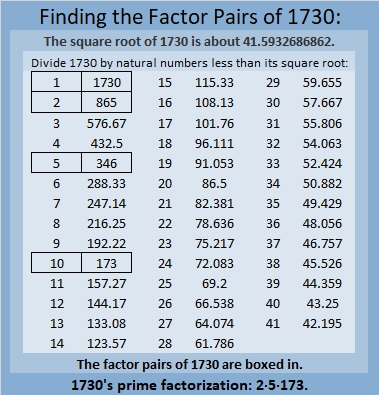### More about the number 1730:

1730 is the sum of two squares in two different ways:
41² + 7² = 1730, and
37² + 19² = 1730.

1730 is the hypotenuse of FOUR Pythagorean triples:
520 1650 1730 which is 10 times (52-165-173)
574 1632 1730 calculated from 2(41)( 7), 41² – 7², 41² + 7²,
1008 1406 1730 calculated from 37² – 19², 2(37)(19), 37² + 19², and
1038 1384 1730 which is 346 times (3-4-5).

Finally, OEIS.org informs us that 1730 is the sum of consecutive squares in two different ways. What are those two ways? I figured it out. Can you?

Here’s a hint: It is the sum of three consecutive squares as well as twelve consecutive squares. That means √(1730/3) rounded is included in one sum and √(1730/12) rounded is included in the other. The solution can be found in the comments. Have fun finding them yourself though!

# 1729 Not a Dull Number

### Today’s Puzzle:

A little more than a hundred years ago near Cambridge University G. H. Hardy took a taxi to visit his young friend and fellow mathematician, Srinivasa Ramanujan, in the hospital. Hardy couldn’t think of anything interesting about his taxi number, 1729, and remarked to Ramanujan that it appeared to be a rather dull number. But even the reason for his hospitalization could not prevent Ramanujan’s genius from shining through. He immediately recognized 1729’s unique and very interesting attribute: it is the SMALLEST number that can be written as the sum of two cubes in two different ways! Indeed,
12³ + 1³ = 1729, and
10³ + 9³ = 1729.

Today’s puzzle looks a little bit like a modern-day American taxi cab with the clues 17 and 29 at the top of the cab. The table below the puzzle contains all the Pythagorean triples with hypotenuses less than 100 sorted by legs and by hypotenuses. Use the table and logic to write the missing sides of the triangles in the puzzle. The right angle on each triangle is the only one that is marked. Obviously, none of the triangles are drawn to scale.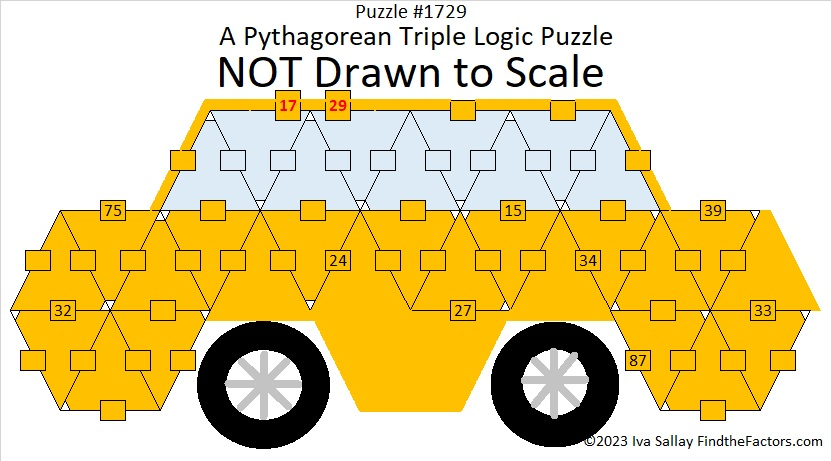Here’s the same puzzle without all the added color: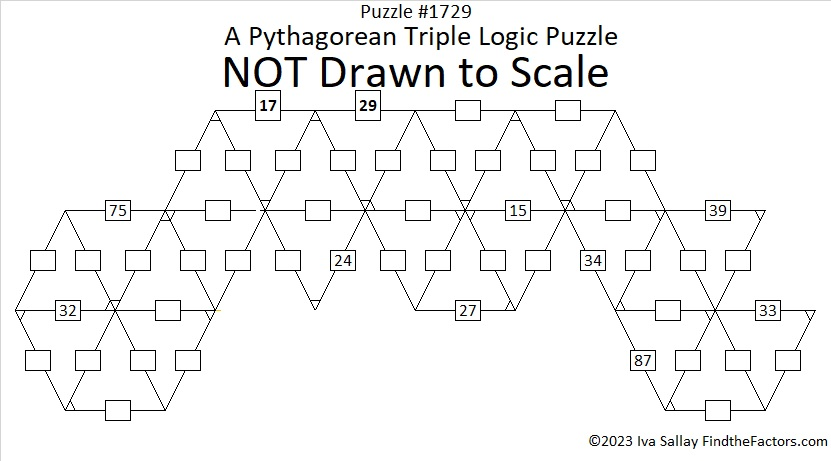Print the puzzles or type the solutions in this excel file: 10 Factors 1721-1729.

What taxi cab might Hardy have tried to catch next? He might have had to wait a long time for it, 4104.
16³ + 2³ = 4104, and
15³ + 9³ = 4104.

### Factors of 1729:

• 1729 is a composite number.
• Prime factorization: 1729 = 7 × 13 × 19.
• 1729 has no exponents greater than 1 in its prime factorization, so √1729 cannot be simplified.
• The exponents in the prime factorization are 1, 1, and 1. Adding one to each exponent and multiplying we get (1 + 1)(1 + 1)(1 + 1) = 2 × 2 × 2 = 8. Therefore 1729 has exactly 8 factors.
• The factors of 1729 are outlined with their factor pair partners in the graphic below.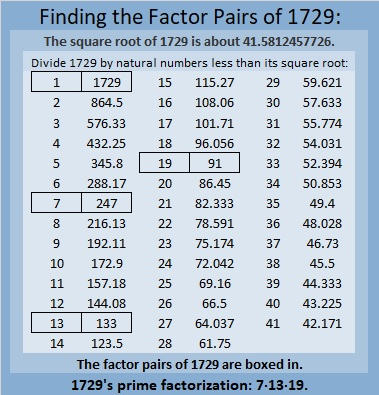### More About the Number 1729:

Did you notice these cool-looking factor pairs?
19 · 91 = 1729.
13 · 133 = 1729.

1729 is the hypotenuse of a Pythagorean triple:
665-1596-1729 which is 133 times (5-12-13).

1729 is the difference of two squares in four different ways:
865² – 864² = 1729,
127² – 120² = 1729,
73² – 60² = 1729, and
55² – 36² = 1729.

# 1728 Is a Perfect Cube

### Today’s Puzzle:

I think this puzzle is a little bit harder than the previous two puzzles, so you might want to solve those two first. You will need to write all the numbers from 1 to 24 where the factors go, but keep the numbers from 1 to 12 together and the numbers from 13 to 24 together. There is only one solution. The possible factors are written to the right of the puzzle. Good luck!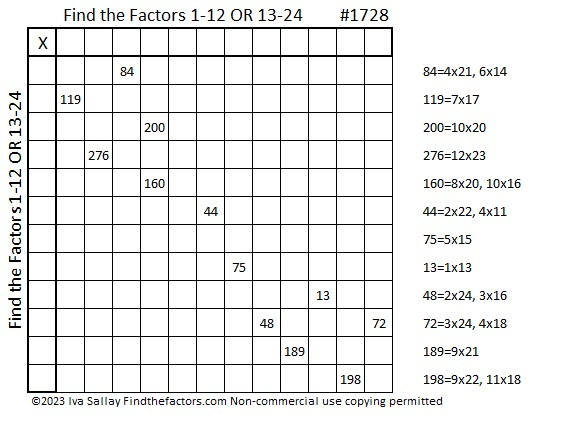You can solve the puzzle by eliminating factors that must be used for other numbers. For example, 198 cannot be 9×22 because 189 must be 9×21, and the puzzle can only have one nine. Don’t write the factors on the multiplication table until after you know if the top row has the numbers 1 – 12 or if it has the numbers 13 – 24. The first column will then have the other set of numbers.

### Factors of 1728:

Let’s find out the factoring information of the puzzle number:

• 1728 is a composite number and a perfect cube.
• Prime factorization: 1728 = 2 × 2 × 2 × 2 × 2 × 2 × 3 × 3 × 3, which can be written 1728 = 2⁶ × 3³.
• 1728 has at least one exponent greater than 1 in its prime factorization so √1728 can be simplified. Taking the factor pair from the factor pair table below with the largest square number factor, we get √1728 = (√576)(√3) = 24√3.
• The exponents in the prime factorization are 6 and 3. Adding one to each exponent and multiplying we get (6 + 1)(3 + 1) = 7 × 4 = 28. Therefore 1728 has exactly 28 factors.
• The factors of 1728 are outlined with their factor pairs in the graphic below.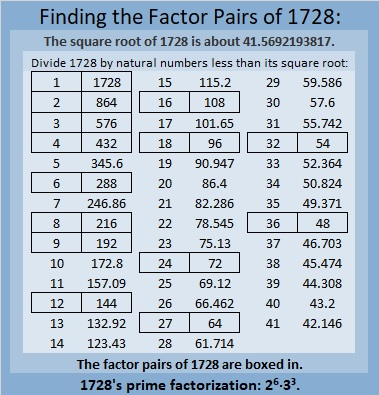### More About the Number 1728:

1728 is the 3rd smallest number with exactly 28 factors.

There are 1728 cubic inches in one cubic foot.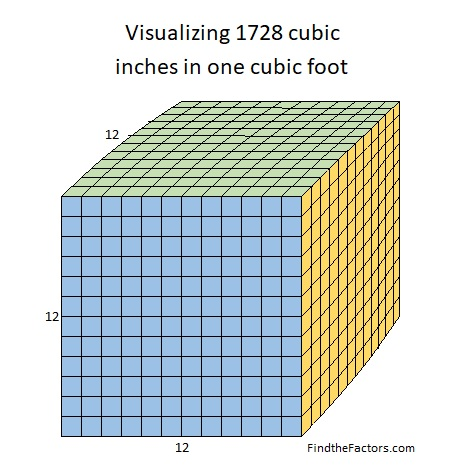12³₁₀ = 10³₁₂ (The cube above is 12 cubed in base 10, and it’s 10 cubed in base 12.)

1728 can be written as the sum of powers in some interesting ways:

2¹⁰ + 2⁹ + 2⁷ + 2⁶ = 1024 + 512 + 128 + 64 = 1728.
1(4⁵) + 2(4⁴) + 3(4³) = 1728.
1(6⁴) + 2(6³) = 1728.
3(8³) + 3(8²) = 3(8³ + 8²) = 1728.

11³ = 1331, and 1(11³) + 3(11²) + 3(11¹) + 1(11⁰) = 1728.

Here’s another connection with the number 11.
3(11²) = 363, and
1728 is palindrome 363 in base 23 because
3(23²) + 6(23¹) + 3(23⁰) = 1728.

1728 is 300 in base 24 because 3(24²) = 1728.

I’ve enjoyed researching the number 1728, and I hope you’ve learned some new and interesting fact about it today.

# 1727 A Happy Birthday Cake for Jo Morgan

### Today’s Puzzle:

Today is Jo Morgan’s birthday, so I made her a cake!

Use logic to find the factors in the cake. There is only one solution. Yesterday’s puzzle was pretty easy at least to get started. This one won’t be. You’ll have to use logic just to figure out which set of numbers belongs in the top row, 1-12 OR 13-24, and which set belongs in the first column. Not only that but both 126 and 180 appear THREE times in the puzzle! Have a party figuring it out!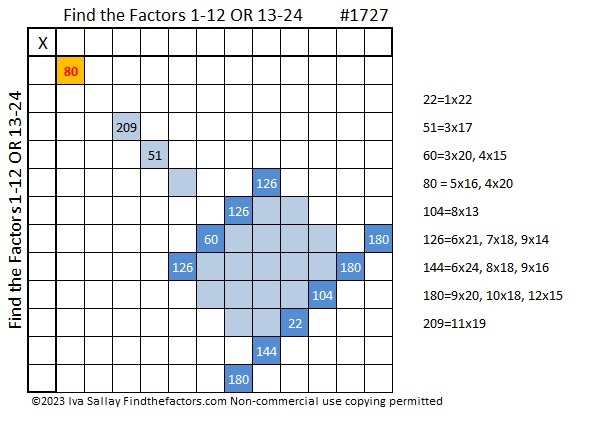### Factors of 1727:

1 – 7 + 2 – 7 = -11, a multiple of 11, so 1727 is divisible by 11.

• 1727 is a composite number.
• Prime factorization: 1727 = 11 × 157.
• 1727 has no exponents greater than 1 in its prime factorization, so √1727 cannot be simplified.
• The exponents in the prime factorization are 1 and 1. Adding one to each exponent and multiplying we get (1 + 1)(1 + 1) = 2 × 2 = 4. Therefore 1727 has exactly 4 factors.
• The factors of 1727 are outlined with their factor pair partners in the graphic below.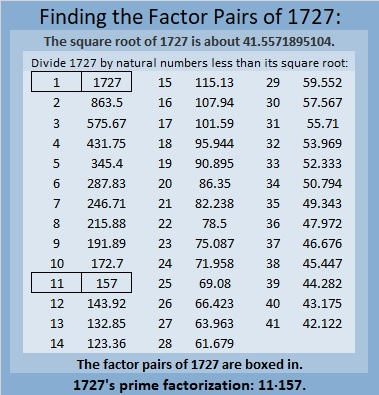### More About the Number 1727:

1727 is the hypotenuse of a Pythagorean triple:
935-1452-1727, which is 11 times (85-132-157).

From OEIS we learn that
1727 = 12³ – 1³, and
7271 = 20³ – 9³.
Thus 1727 forward and backward is the difference of two cubes!

# 1726 Find the Factors 1-12 AND 13-24

### Today’s Puzzle:

Here’s a puzzle I made to start off 2023. Either all the numbers from 1 to 12 will go in the top row OR they will all go in the first column. All the numbers from 13 to 24 will go in the remaining area. The possible factors are written on the side of the puzzle. Can you find all the factors? There is only one solution.### Factors of 1726:

• 1726 is a composite number.
• Prime factorization: 1726 = 2 × 863.
• 1726 has no exponents greater than 1 in its prime factorization, so √1726 cannot be simplified.
• The exponents in the prime factorization are 1 and 1. Adding one to each exponent and multiplying we get (1 + 1)(1 + 1) = 2 × 2 = 4. Therefore 1726 has exactly 4 factors.
• The factors of 1726 are outlined with their factor pair partners in the graphic below.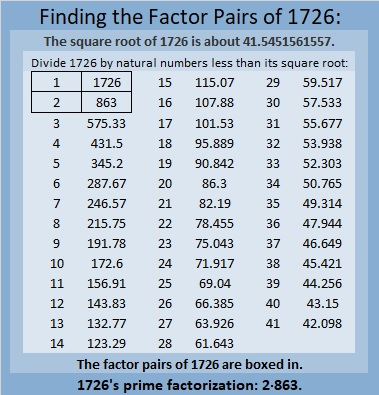### More About the Number 1726:

1726 is the sum of four consecutive numbers from 430 to 433.

# 1725 A Toast to You on This Last Day of the Year

### Today’s Puzzle:

I haven’t posted as much this past year as I ought, but thank you for bearing with me. Here is a toast to you, faithful reader! Here’s hoping for a great new year for all of us! Write the numbers from 1 to 10 in both the first column and the top row so that the given clues are the products of the factors you write.### Factors of 1725:

• 1725 is a composite number.
• Prime factorization: 1725 = 3 × 5 × 5 × 23, which can be written 1725 = 3 × 5² × 23.
• 1725 has at least one exponent greater than 1 in its prime factorization so √1725 can be simplified. Taking the factor pair from the factor pair table below with the largest square number factor, we get √1725 = (√25)(√69) = 5√69.
• The exponents in the prime factorization are 1, 2, and 1. Adding one to each exponent and multiplying we get (1 + 1)(2 + 1)(1 + 1) = 2 × 3 × 2 = 12. Therefore 1725 has exactly 12 factors.
• The factors of 1725 are outlined with their factor pair partners in the graphic below.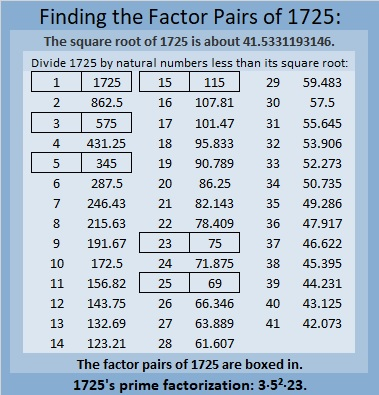### More About the Number 1725:

1725 is the difference of two squares in six different ways:

863² – 862² = 1725,
289² – 286² = 1725,
175² – 170² = 1725,
65² – 50² = 1725,
49² – 26² = 1725, and
47² – 22² = 1725.